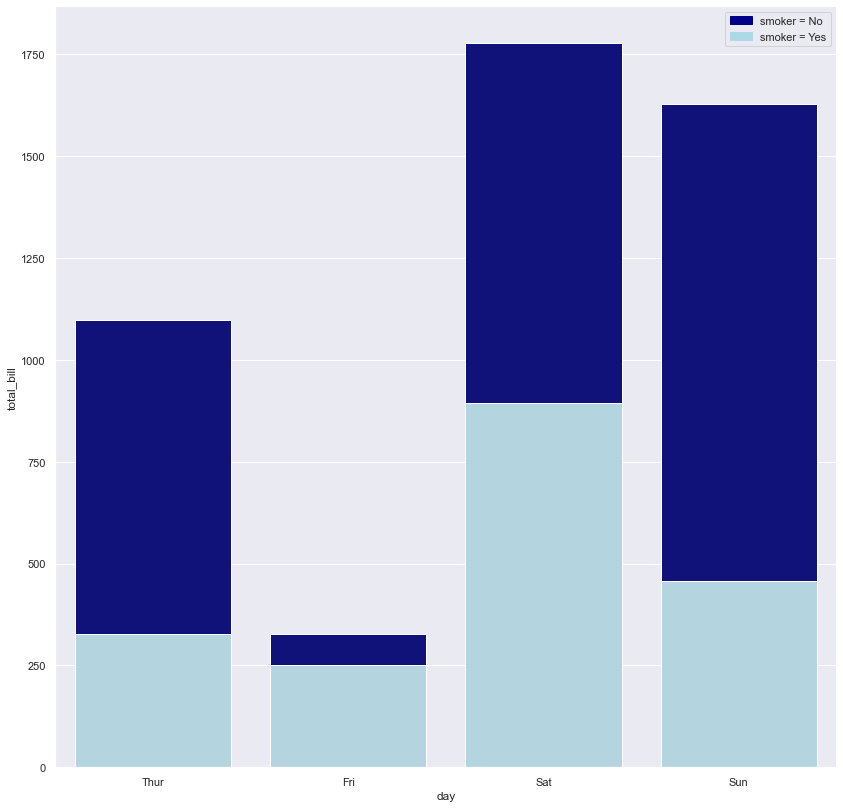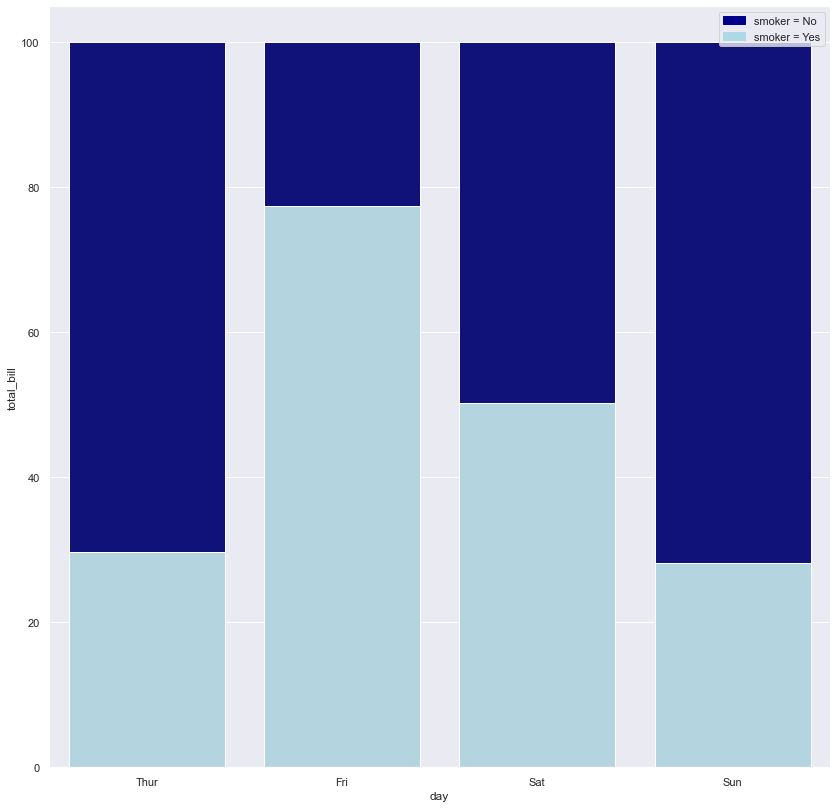## Stacked Barplot

In stacked barplot, subgroups are displayed as bars on top of each other. Although `barplot()` function doesn't have a parameter to draw stacked bars, you can plot a stacked bar chart by putting the bar charts on top of each other like in the example below:

``````# import libraries
import seaborn as sns
import numpy as np
import matplotlib.pyplot as plt
import matplotlib.patches as mpatches

# set plot style: grey grid in the background:
sns.set(style="darkgrid")

# set the figure size
plt.figure(figsize=(14, 14))

# top bar -> sum all values(smoker=No and smoker=Yes) to find y position of the bars
total = tips.groupby('day')['total_bill'].sum().reset_index()

# bar chart 1 -> top bars (group of 'smoker=No')
bar1 = sns.barplot(x="day",  y="total_bill", data=total, color='darkblue')

# bottom bar ->  take only smoker=Yes values from the data
smoker = tips[tips.smoker=='Yes']

# bar chart 2 -> bottom bars (group of 'smoker=Yes')
bar2 = sns.barplot(x="day", y="total_bill", data=smoker, estimator=sum, ci=None,  color='lightblue')

top_bar = mpatches.Patch(color='darkblue', label='smoker = No')
bottom_bar = mpatches.Patch(color='lightblue', label='smoker = Yes')
plt.legend(handles=[top_bar, bottom_bar])

# show the graph
plt.show()``````## Percent Stacked Bar Chart

In percent stacked chart, bars are again displayed on top of each other, and they represent the proportion of each subgroup in the whole.

``````# import libraries
import seaborn as sns
import numpy as np
import matplotlib.pyplot as plt
import matplotlib.patches as mpatches

# set the figure size
plt.figure(figsize=(14, 14))

# from raw value to percentage
total = tips.groupby('day')['total_bill'].sum().reset_index()
smoker = tips[tips.smoker=='Yes'].groupby('day')['total_bill'].sum().reset_index()
smoker['total_bill'] = [i / j * 100 for i,j in zip(smoker['total_bill'], total['total_bill'])]
total['total_bill'] = [i / j * 100 for i,j in zip(total['total_bill'], total['total_bill'])]

# bar chart 1 -> top bars (group of 'smoker=No')
bar1 = sns.barplot(x="day",  y="total_bill", data=total, color='darkblue')

# bar chart 2 -> bottom bars (group of 'smoker=Yes')
bar2 = sns.barplot(x="day", y="total_bill", data=smoker, color='lightblue')

top_bar = mpatches.Patch(color='darkblue', label='smoker = No')
bottom_bar = mpatches.Patch(color='lightblue', label='smoker = Yes')
plt.legend(handles=[top_bar, bottom_bar])

# show the graph
plt.show()``````## Contact & Edit

👋 This document is a work by Yan Holtz. You can contribute on github, send me a feedback on twitter or subscribe to the newsletter to know when new examples are published! 🔥